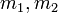# Newton's third law

(diff) ← Older revision | Latest revision (diff) | Newer revision → (diff)
QUICK PHRASES: action and reaction are equal and opposite

## Statement

Newton's third law or Newton's third law of motion states that if one body$A$ exerts a force on another body$B$, the other body exerts a force that is equal in magnitude and opposite in direction to that exerted by$A$ on$B$.

The pair of these two forces is termed an action-reaction pair, and each of the forces can be termed the reaction to the other force. Newton's third law is thus often stated as action and reaction are equal and opposite.

Note that in Newton's third law, action and reaction play a similar role, and the reaction force does not occur after or in response to the action force in any meaningful sense.

## Particular cases

Type of force Pair of bodies Directions of the action-reaction pair Magnitude of the action-reaction pair
Gravitational force Two masses that exert a gravitational force on each other. In most earthly situations, one of the bodies is the earth. On each body, in the direction toward the center of mass of the other body. Thus the force acts inward on both bodies along the line joining the centers of mass of the two bodies$Gm_1m_2/r^2$ where$G$ is the gravitational constant,$m_1, m_2$ are the masses and$r$ is the distance between the centers of mass
Normal force Bodies that share a surface of contact. On each body, the force acts in the outward direction along the surface of contact. The magnitude is determined to be such that the net relative acceleration along the direction perpendicular to the surface of contact is zero. It thus adjusts in response to the other forces being exerted on the bodies.
Static friction Bodies that share a surface of contact. On each body, the force acts in a direction parallel to the surface of contact, opposing the tendency of slippage of surfaces. The magnitude is determined so that the net relative acceleration of surfaces along the surface of contact is zero. It thus adjusts in response to the other forces being exerted on the bodies. There is, however, an upper limit: the limiting coefficient of static friction times the normal force magnitude.
Kinetic friction Bodies that share a surface of contact. On each body, the force acts in a direction parallel to the surface of contact, opposing the actual direction of slippage of surfaces. The magnitude is a fixed multiple of the normal force, where the constant of proportionality is the coefficient of kinetic friction and is determined by the nature of the surfaces in contact.
Tension An inextensible string and a body attached to one end of the string The string pulls the body inward; the body pulls the string outward. Determined from other constraints.